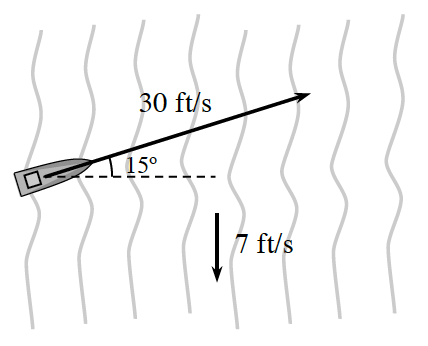### Home > PC3 > Chapter 6 > Lesson 6.2.3 > Problem6-109

6-109.A boat is headed across a river at an angle of $15^\circ$ with a rate of $30$ feet per second. The current is heading down stream at a rate of $7$ feet per second. The river is $1000$ feet wide.

1. Express the velocity of the boat as a vector in component form without including the effect of the river.

Sketch and label a right triangle for the given situation.
Use cosine and sine to determine the horizontal and vertical components.

2. The actual motion of the boat is the combination of the motion of the boat and the current in the river. What is the vector that gives the actual motion of the boat?

The vector for the current is $⟨0, −7⟩.$
Use vector addition to determine the resultant vector.

3. How long will it take the boat to cross the river?

Consider the horizontal component of the resultant vector from part (b).

4. How far up river will the boat arrive?

Consider the vertical component of the resultant vector from part (b).Multiply this by the number of seconds found in part (c).